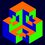# Homework is given to a child who is not ready for a math competition - (2).

My teacher gave me this problem:

$$a$$, $$b$$ and $$c$$ are three positives such that $$abc = 1$$. Prove that

$\large M = \dfrac{1}{a^2 - a + 1} + \dfrac{1}{b^2 - b + 1} + \dfrac{1}{c^2 - c + 1} \le 3$

Here's how I solve it.

\large \begin{aligned} M &= \dfrac{1}{a^2 - a + 1} + \dfrac{1}{b^2 - b + 1} + \dfrac{1}{c^2 - c + 1}\\ &= \left(a + 1 - \dfrac{a^3}{a^2 - a + 1}\right) + \left(b + 1 - \dfrac{b^3}{b^2 - b + 1}\right) + \left(c + 1 - \dfrac{c^3}{c^2 - c + 1}\right) && m^3 + 1 = (m + 1)(m^2 - m + 1)\\ &= (a + b + c) - \left(\dfrac{a^2}{bc + a - 1} + \dfrac{b^2}{ca + b - 1} + \dfrac{c^2}{ab + c - 1}\right) + 3 && abc = 1\\ &\le (a + b + c) - \dfrac{(a + b + c)^2}{(ab + bc + ca) + (a + b + c) - 3} + 3 && \text{Sedrakyan's inequality, Engel’s form or Titu’s lemma}\\ &\le (a + b + c) - \dfrac{(a + b + c)^2}{\dfrac{(a + b + c)^2}{3} + (a + b + c) - 3} + 3 && ab + bc + ca \le a^2 + b^2 + c^2\\ \end{aligned}

(Fun fact, the Sedrakyan's inequality is called the Schwarz inequality here.)

Let $a + b + c = n$, we have that $a + b + c \ge 3\sqrt{abc} = 3$ and $M \le n - \dfrac{3n^2}{n^2 + 3n - 9} + 3$.

The problem becomes:

Calculate the maximum value of the following expression if $n \ge 3$.

$\large M' = n - \dfrac{3n^2}{n^2 + 3n - 9} + 3$

So if you can:

• solve the next part of the solution

• (prove that there is no maximum value of $M'$ and) solve the original problem in a faster, more convenient wayNote by Thành Đạt Lê
2 years, 6 months ago

This discussion board is a place to discuss our Daily Challenges and the math and science related to those challenges. Explanations are more than just a solution — they should explain the steps and thinking strategies that you used to obtain the solution. Comments should further the discussion of math and science.

When posting on Brilliant:

• Use the emojis to react to an explanation, whether you're congratulating a job well done , or just really confused .
• Ask specific questions about the challenge or the steps in somebody's explanation. Well-posed questions can add a lot to the discussion, but posting "I don't understand!" doesn't help anyone.
• Try to contribute something new to the discussion, whether it is an extension, generalization or other idea related to the challenge.

MarkdownAppears as
*italics* or _italics_ italics
**bold** or __bold__ bold
- bulleted- list
• bulleted
• list
1. numbered2. list
1. numbered
2. list
Note: you must add a full line of space before and after lists for them to show up correctly
paragraph 1paragraph 2

paragraph 1

paragraph 2

[example link](https://brilliant.org)example link
> This is a quote
This is a quote
    # I indented these lines
# 4 spaces, and now they show
# up as a code block.

print "hello world"
# I indented these lines
# 4 spaces, and now they show
# up as a code block.

print "hello world"
MathAppears as
Remember to wrap math in $$ ... $$ or $ ... $ to ensure proper formatting.
2 \times 3 $2 \times 3$
2^{34} $2^{34}$
a_{i-1} $a_{i-1}$
\frac{2}{3} $\frac{2}{3}$
\sqrt{2} $\sqrt{2}$
\sum_{i=1}^3 $\sum_{i=1}^3$
\sin \theta $\sin \theta$
\boxed{123} $\boxed{123}$

Sort by:

Well, the expression is in one variable 'n' and also, we know from the given condition of abc=1 that a+b+c = n is atleast 3......(AM-GM)........Now, by simple first derivative,we see that the expression in M is always increasing for n>=3..........And hence, the maximum value of M is 3 which occurs when n = 3 or a+b+c=3 or a=b=c=1.........!!! Here is a graph supporting the answer..........

Also, my approach was on the same lines as yours........I usually try to reduce inequalities to a single variable, just like you have done.....!!

- 2 years, 6 months ago

I am really sorry but I am just in grade 9 so I can't use derivatives, I hope that you can come up a solution completely in pure algebra.

- 2 years, 6 months ago

Now, by simple first derivative,we see that the expression in M is always increasing for n>=3

Care to elaborate? I don't see the connection between the Desmos graph and the inequality posed.

- 2 years, 6 months ago

Here Sir, I have added the inequality of the derivative, showing the region where the function is increasing...........Hope it is clear now......:)

- 2 years, 6 months ago

M is always increasing for n>=3..........And hence, the maximum value of M is 3 which occurs when n = 3 or a+b+c=3 or a=b=c=1.........!!!

Your first statement claims that $M$ has no upper bound. But your second statement says it has an upper bound of 3. How is that possible?

- 2 years, 6 months ago